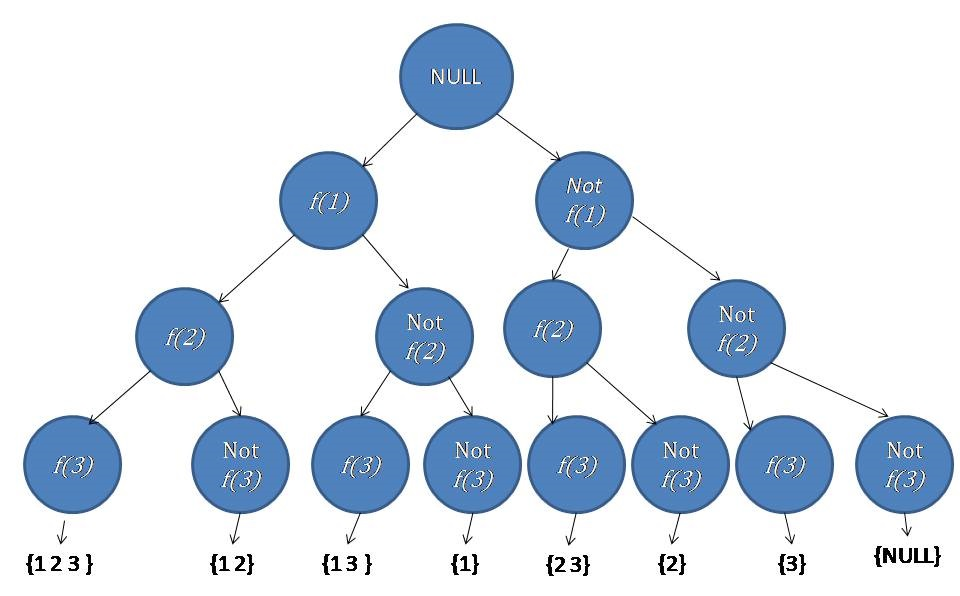Q:

# Backtracking to find all subsets

There is a set contains N number of elements. You have to find out all the possible subsets of the given set and print them.

```    Input:
Test case T
//T no. of lines with the value of N and corresponding values.
E.g.
2

4
1 2 3 4

2
7 8

1<=T<=100
1<=N<=1000

Output:
Print the subsets of the set.
```

Example

```    T=2

Input:
N=4
1 2 3 4

Output:
1 2
1 2 3
1 2 3 4
1 2 4
1 3
1 3 4
1 4
2
2 3
2 3 4
2 4
3
3 4
4

Input:
N=2
7 8

Output:
7
7 8
8```

Let, there is a Set S having N number of elements,

```    S = {X1, X2, X3, ..., Xn}
```

The process to print the subsets of the set is a problem of combination and permutation. To get the result we use the backtracking process.

```    Let,
f(i) = function to insert the ith number into a subset.
```

Here, we take a subset of that set in our consideration and consider two things,

1. An element is a part of that subset ( f(i) ).
2. An element is not a part of that subset ( not f(i) ).
```    For: N=3
S = { 1 2 3 }
```Algorithm:

Here we use the vector STL to store the subsets.

```    traverse(arr, n, current_pos,set,subset){
if(Current_pos is greater or equals to the n)Then
return
end if
for i = current_pos to the end of the set
insert the element of arr[i] into subset
insert the subset into the set
traverse(arr,n,i+1,set,subset)
pop the element from subset
end for
}
```

C++ implementation:

``````#include <bits/stdc++.h>
using namespace std;

void traverse(int* arr, int n, int pos, vector<vector<int> >& v, vector<int> vi)
{
//if the current position is greater than or equals to n
if (pos >= n)
return;
for (int i = pos; i < n; i++) {
vi.push_back(arr[i]);
v.push_back(vi);
traverse(arr, n, i + 1, v, vi);
vi.pop_back();
}
}

vector<vector<int> > find_subset(int* arr, int n)
{
vector<vector<int> > v;
vector<int> vi;
int pos = 0;
traverse(arr, n, pos, v, vi);
return v;
}

void print(vector<vector<int> > v)
{
for (int i = 0; i < v.size(); i++) {
for (int j = 0; j < v[i].size(); j++) {
cout << v[i][j] << " ";
}
cout << endl;
}
}

int main()
{
int t;

cout << "Test Case : ";
cin >> t;

while (t--) {
int n;

cout << "Enter the value of n : ";
cin >> n;

int arr[n];

//taking the set elements
cout << "Enter the values : ";
for (int i = 0; i < n; i++) {
cin >> arr[i];
}

vector<vector<int> > v = find_subset(arr, n);

//print the vector
print(v);
}
return 0;
}``````

Output

```Test Case : 2
Enter the value of n : 4
Enter the values : 1 2 3 4
1
1 2
1 2 3
1 2 3 4
1 2 4
1 3
1 3 4
1 4
2
2 3
2 3 4
2 4
3
3 4
4
Enter the value of n : 2
Enter the values : 7 8
7
7 8
8```